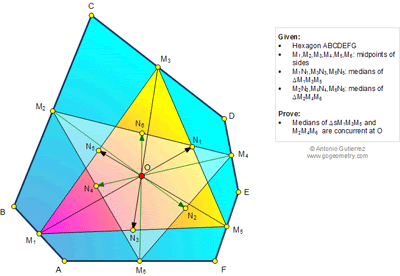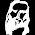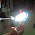## Wednesday, April 23, 2014

### Geometry Problem 1007: Hexagon, Midpoint, Triangle, Median, Centroid, Concurrency

Level: Mathematics Education, High School, Honors Geometry, College.

Click the figure below to see the complete problem 1007.1.Using mass point approach.

Let all points in the figure have mass 1.

Then
2M₁=A+B
2M₂=B+C
2M₃=C+D
2M₄=D+E
2M₅=E+F
2M₆=F+A

Let O₁ and O₂ be the centroid of ΔM₁M₃M₅ and ΔM₂M₄M₆, thus
3O₁=M₁+M₃+M₅, i.e. 6O₁=A+B+C+D+E+F
3O₂=M₂+M₄+M₆, i.e. 6O₂=A+B+C+D+E+F

Hence, O₁=O₂=O, which is the intersection of six medians.

2.Let V(X) represent position vector of point X
We have V(M1)= ½(V(A)+V(B))
Similarly we also have V(M2) and V(M3)
Since O is the centroid of triangle M1M3M5
So V(O)=1/3(V(M1)+V(M3)+V(M5))= 1/6 ( V(A)+ V(B)+ V(C)+ V(D)+ V(C)+ V(F))
Similarly we have V(M2), V(M4) and V(M6)
And vector of centroid of triangle M2M4M6 will be the same as V(O)

3.I really don’t like the notation in this question, so bear with me as I change the names of the points.
Question (reworded): There is hexagon ABCDEF. L is midpoint of line AF, G is midpoint of line BA, H is midpoint of line CB, I is midpoint of line DC, J is midpoint of line ED, K is midpoint of line FE. Do the medians of triangle LHJ concur with medians of Triangle GIK?
We use Barycentric Coordinates.
Definition: Capital letters will denote vectors. P = x L + y H + z J will be written as P = (x, y, z). By definition, x + y + z = 1. AB means “length of AB” unless otherwise specified; most importantly, it does NOT mean “A times B”. If Z is midpoint of X, Y, then Z = (1/2)(X + Y).
The intersection of medians is the centroid. That the centroid exists is trivially proven with Ceva’s theorem. The centroid of Triangle LHJ is (1/3, 1/3, 1/3); this is trivial to prove, but for those who want a proof: the equation of the median from L is y = z as this linear equation satisfies (1, 0, 0) and (0, ½, ½) which is midpoint of H, J. Similarly, the equation of the median from H is x = z. Given that x + y + z = 1, it’s obvious that the intersection occurs at (1/3, 1/3, 1/3).
In other words, the centroid of LHJ is the average of L, H, J. Similarly, the centroid of Triangle GIK is (1/3)(G + I + K).
As G, I, K are midpoints of line AB, line CD and line EF, it’s obvious that the centroid of Triangle GIK is (1/6)(A + B + C + D + E + F), which, when rearranged, makes (1/3)([A + F]/2 + [E + D]/2 + [C + B]/2). It’s known that L, K, J are midpoints of line AF, line BC and line DE. Hence, (1/3)([A + F]/2 + [E + D]/2 + [C + B]/2) = (1/3)(L + H + J) = (1/3, 1/3, 1/3).
We have proved that the centroid of triangle LHJ is the same point as the centroid of triangle GIK. Hence, all the medians concur.